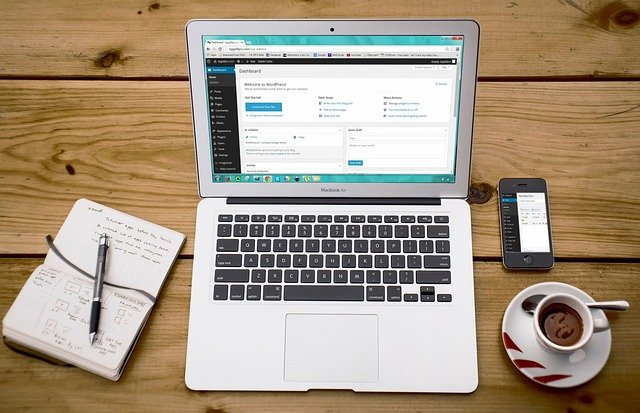Welcome to DigiStacKedu##### Does R Programming is required to be Data Analyst. ?

R is a very popular programming language used by many companies across the globe and it is developed by Robert Gentleman, provides statistical computing techniques for data analysis and data visualization. R professionals work on R statistical methods such as classification, clustering, machine learning techniques, and various statistical tests. R programming also provides packages for data manipulation and data visualization such as are ggplot, and plotly.

Thousands of data scientists use R Programming for data analysis and data mining, it also gives a lot of job opportunities for the students and professionals.

Is R is required for Data Science.?

The Data Science field covers concepts such as data cleaning, statistical techniques, probability, machine learning, and data visualization and these concepts can be learned using python or R programming language, companies using python for data analysis but some of them also using R programming for the same. it is not mandatory to learn R but if any company job profile is demanding then you must have knowledge of the R programming language.

Scope of R Programming.

1. R Programming Scope For Business

R programming saves analysis cost and time as r is easy to learn and coders write less code compared to other programming languages moreover, it is an open-source project that is why R programming is the first choice for many organizations and also gives functionality to connect with various database management systems and provide more than 5000 packages for data analysis.

2. R Programming Scope for Students

R programming is easy to learn and gives advanced graphical charts for data visualization and also provides a lot of opportunities for students and professionals. I will advise you to learn R and python both to be a data scientist. you should learn R statistical concepts like how to calculate mean, median, standard deviation, and how to work on R graphics like scatterplots, histograms, and heatmap.

3. R Programming Scope for Statistical Learning

Starting a data science career can become a challenge for nonprogrammers. The advantage of R is that it provides statistical analysis using a programming language even you can create a custom solution by user-defined functions moreover, anyone can use it and extend functionality by writing new packages. statistical analysis includes Sampling Distribution, Hypothesis Testing, ANOVA, Chi-Square, T-Test, etc.

4. R programming Scope in Data Analysis

The first point to begin analysis is getting the knowledge of data analysis. This is not all about only R programming but it requires knowledge of probability, descriptive and inferential statistics that is why I would suggest you learn statistics before learning R programming Language. You must try our best R programming course in which we will cover statistics and R programming. .

Machine Learning using R

R is a tool for analyzing and manipulating data and experts use R programming to create machine learning predictive models that help to make decisions for the organizations. R packages tidyverse, dplyr, tidyr, and ggplot2 are used for data manipulation moreover there are various predefined models such as regression, naive Bayes, classification trees, and support vector machine for data analysis.

How I can make career in Data Science.

1. Learn R programming

R provides a statistical analysis package, it is open-source software and anyone can use and change it. R is good for GNU/Linux and Microsoft Windows. moreover, it is having a cross-platform which runs on many operating systems. I would suggest you should learn R programming if you want to make your career in Data Science.

2. Learn Data Analysis

Data analysis is the process in which we analyze different varieties of data. These data might include census, survey, and scientific data. most of the experts analyzing the huge amounts of data collected through transactions within companies and websites. Data analysis includes analysis, capture, filter, cleaning, storage, visualization, and privacy.

3. Learn R GGplot

Data Visualization is an essential part and the skill of data analysis in R . It provides various packages to implement data visualization. The most popular is the ggplot2. this package is used for data visualization and exploratory data analysis moreover, using this library one can detect relationships between variables. we create different types of charts in order to analyze data such as bar charts, heatmap, music charts, bubble charts, etc.

4. Learn Tableau

Tableau is the most popular software for business intelligence. It can access data from a variety of sources like SQL, Hadoop, Excel, Oracle, and JSON. It can combine varieties of data and using simple drag and drop operations moreover, tableau gives us the functionality to draw various analytical charts like histograms, pie charts, line charts, regression lines, etc. most of the companies using table because it is designed to built-in visual practices and data exploration.